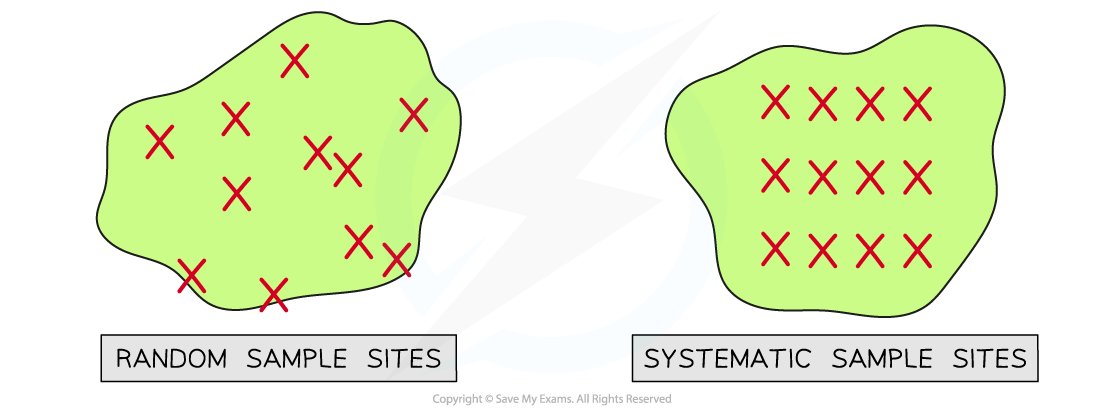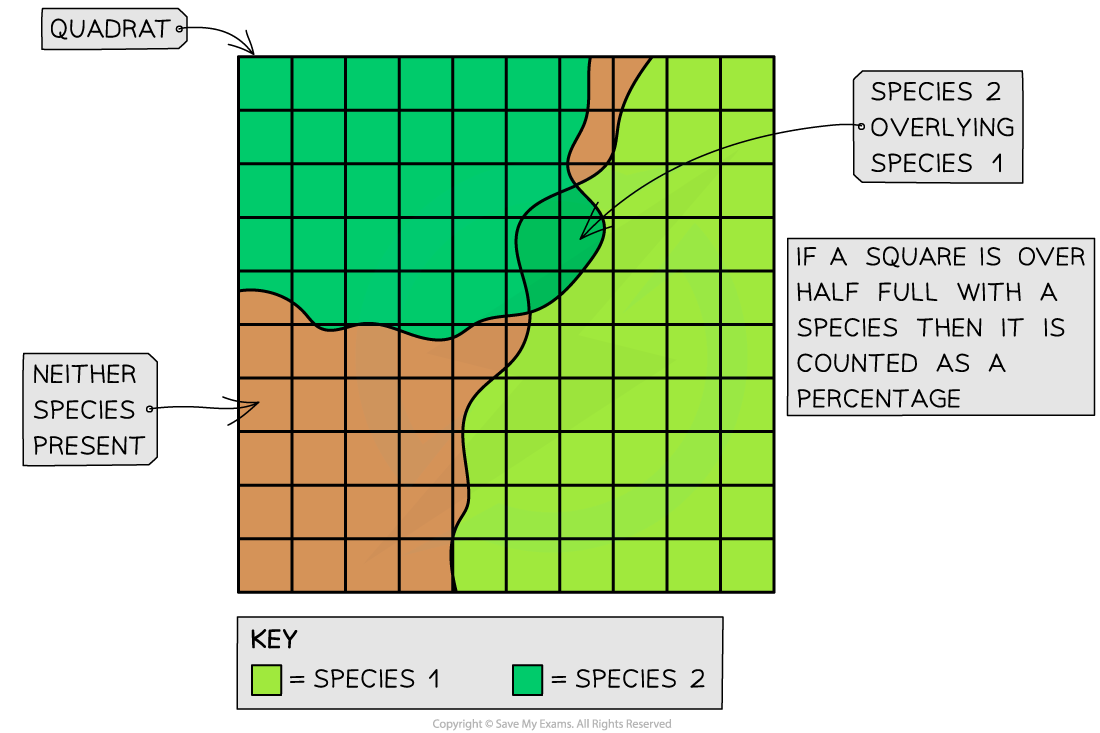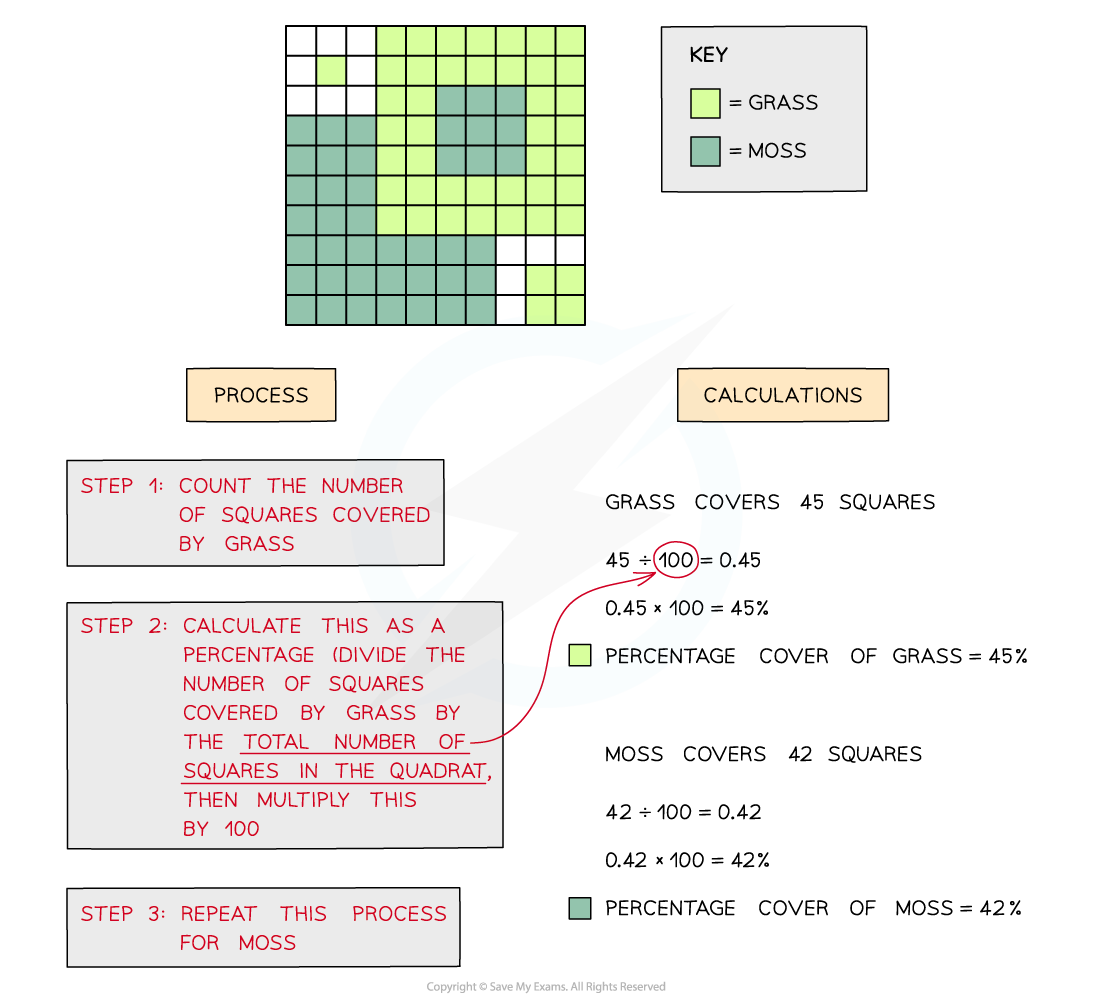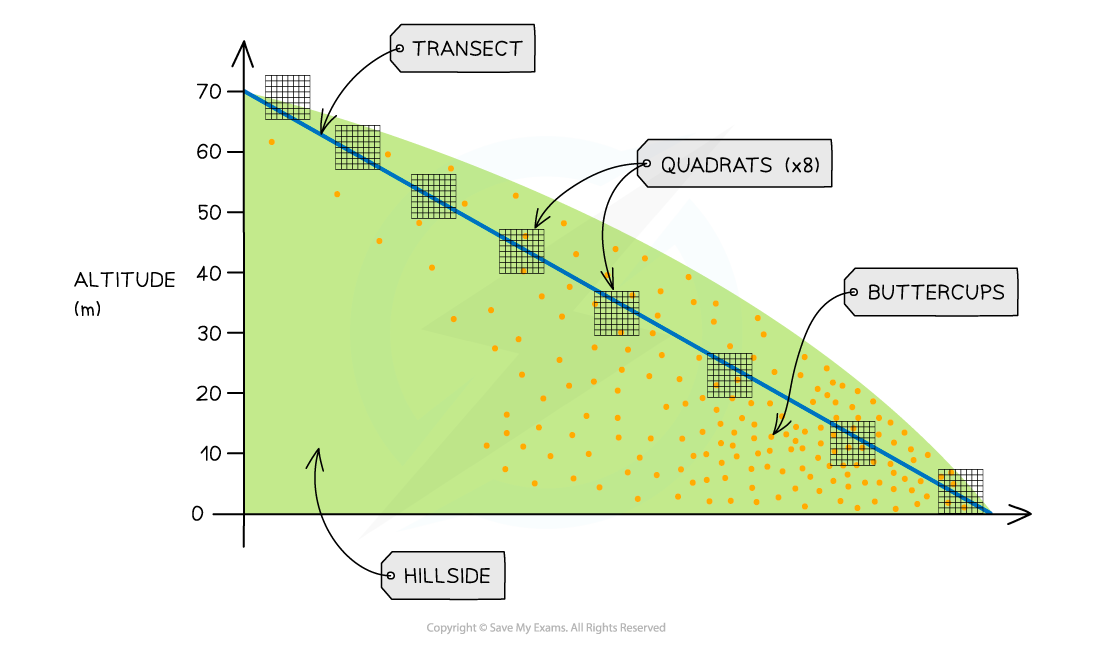# AQA A Level Biology复习笔记7.4.4 Estimating the Size of a Population

### Estimating the Size of a Population

• Measuring all the different levels of biodiversity within an ecosystem could be very time consuming
• Finding out which species live in an ecosystem and the size of the populations requires the identification and cataloguing of all organisms present to build a species list
• This is possible for areas that are very small or where the species are very large like trees
• However, for larger and more complex ecosystems like rainforests, it is simply impossible to find, identify and count every organism that exists there
• When this is the case different samples of the area can be taken and used to make an estimate for the total species numbers in the area

#### Sampling

• Sampling is a method of investigating the abundance and distribution of species and populations
• There are two different types of sampling:
• Random
• Systematic

• In random sampling the positions of the sampling points are completely random or due to chance
• This method is beneficial because it means there will be no bias by the person that is carrying out the sampling that may affect the results

• In systematic sampling the positions of the sampling points are chosen by the person carrying out the sampling
• There is a possibility that the person choosing could show bias towards or against certain areas
• Individuals may deliberately place the quadrats in areas with the least species as these will be easier and quicker to count
• This is unrepresentative of the whole area

• When a sampling area is reasonably uniform or has no clear pattern to the way the species are distributed then random sampling is the best choiceRandom sampling differs from systematic sampling when choosing sample sites on an island

Sampling methods

• There are three main sampling methods used when trying the estimate the size of a population:
• Quadrats (for non-motile or slow-moving species)
• Transects (for non-motile or slow-moving species)
• Mark-release-recapture (for motile species)

### Apparatus & Techniques: Investigating the Distribution of Organisms

• The distribution of a species describes how it is spread throughout the ecosystem
• The abundance of a species is the number of individuals of that species
• The distribution and abundance of non-motile or slow-moving species in an area can be assessed using two different practical methods:
• Belt Transects

• Some ecosystems are very complex with large numbers of different species of different sizes
• For the sake of logistics, sampling is often used to estimate the distribution and abundance of species
• When carrying out sampling, square frames called quadrats can be used to mark off the area being sampled
• Quadrats of different sizes can be used depending on what is being measured and what is most suitable in the space the samples are being made in
• Quadrats must be laid randomly in the area to avoid sampling bias
• This random sampling can be done by converting the sampling area into a grid format and labelling each square on the grid with a number
• Then a random number generator is used to pick the sample points

• Once the quadrat has been laid on the chosen sample point the abundance of all the different species present can be recordedUsing a quadrat to investigate percentage cover of two species of grass. There may be some squares lacking any species and other squares with multiple species - this means the total percentage cover of a single quadrat can sometimes be over or under 100%

• The results from the quadrats can be used to calculate the predicted frequency and density of a species within an area
• Species frequency is the probability that the species will be found within any quadrat in the sample area
• The number of quadrats that the species was present in is divided by the total number of quadrats and then multiplied by 100
• For example, if bluebells were found in 18 out of 50 quadrats the species frequency would be (18/50) x 100 = 36%

• It can sometimes be difficult to count individual plants or organisms. When this is the case percentage cover of the species within the quadrat can be estimated instead
• The quadrat is divided into 100 smaller squares. The number of squares the species is found in is equivalent to its percentage cover in that quadrat
• For example, if grass is found in 89 out of 100 squares in the quadrat then it has a percentage cover of 89%How to estimate percentage cover of one or more species using a quadrat

#### Belt transects

• Throughout some areas, there can be changes in the physical conditions (i.e. abiotic factors)
• For example, there may be changes in altitude, soil pH or light intensity

• When investigating the species distribution in these kinds of areas systematic sampling is more appropriate
• Methods using transects can help show how the distribution or abundance of a species changes with the different physical conditions in the area
• A transect is a line represented by a measuring tape, along which samples are taken

• For a belt transect:
• Place quadrats at regular intervals along the tape and record the abundance of each species within each quadrat
• This produces quantitative dataAn example of a transect on a hillside. A quadrat is placed at regular intervals (every 10m of altitude gained) and counts the number of individuals (of the species being investigated eg. buttercups) in each quadrat

### Apparatus & Techniques: The Mark-Release-Capture Method

#### Mark-release-capture

• The two sampling methods described above are only useful for non-motile (sessile) organisms
• Different methods are required for estimating the number of individuals in a population of motile animals
• The mark-release-capture method is used

• For a single species in the area:
• The first large sample is taken. As many individuals as possible are caught, counted and marked in a way that won’t affect their survival e.g. if studying a species of beetle, a small amount of brightly coloured non-toxic paint can be applied to their carapace (shell)
• The marked individuals are returned to their habitat and allowed to randomly mix with the rest of the population
• When a sufficient amount of time has passed another large sample is captured
• The number of marked and unmarked individuals within the sample are counted
• The proportion of marked to unmarked individuals is used to calculate an estimate of the population size
• The formula for the calculation is:

N = (n1 × n2) ÷ m2

• Where:
• N = population estimate
• n1 = number of marked individuals released
• n2 = number of individuals in the second sample (marked and unmarked)
• m2 = number of marked individuals in the second sample

#### Worked Example

Scientists wanted to investigate the abundance of leafhoppers in a small grassy meadow. They used sweep nets to catch a large sample of leafhoppers from the meadow. Each insect was marked on its underside with non-toxic waterproof paint and then released back into the meadow. The following day another large sample was caught using sweep nets. Use the figures below to estimate the size of the leafhopper population in this meadow.

• No. caught and marked in first sample  (n1) = 236
• No. caught in second sample (n2) = 244
• No. of marked individuals in the second sample (m2)  = 71

Step One: Write out the equation and substitute in the known values

N = (n1 × n2) ÷ m2

N = (236 × 244) ÷ 71

Step Two: Calculate the population size estimate (N)

N = 57,584 ÷ 71

N = 811

#### Results from mark-release-capture

Assumptions

• When using the mark-release-capture method, there are a few assumptions that have to be made:
• The marked individuals are given sufficient time to disperse and mix back in fully with the main population
• The marking doesn't affect the survival rates of the marked individuals (e.g. doesn't make them more visible and therefore more likely to be predated)
• The marking remains visible throughout the sampling and doesn't rub off
• The population stays the same size during the study period (i.e. there are no significant changes in population size due to births, deaths or migrations into or out of the main population)